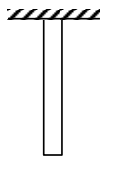# A uniform heavy rod of weight

Question:

A uniform heavy rod of weight $10 \mathrm{~kg} \mathrm{~ms}^{-2}$, crosssectional area $100 \mathrm{~cm}^{2}$ and length $20 \mathrm{~cm}$ is hanging from a fixed support. Young modulus of the material of the rod is $2 \times 10^{11} \mathrm{Nm}^{-2}$. Neglecting the lateral contraction, find the elongation of rod due to its own weight.

1. $2 \times 10^{-9} \mathrm{~m}$

2. $5 \times 10^{-8} \mathrm{~m}$

3. $4 \times 10^{-8} \mathrm{~m}$

4. $5 \times 10^{-10} \mathrm{~m}$

Correct Option: , 4

Solution:We know,

$\Delta \ell=\frac{\mathrm{WL}}{2 \mathrm{AY}}$

$\Delta \ell=\frac{10 \times 1}{2 \times 5} \times 100 \times 10^{-4} \times 2 \times 10^{11}$

$\Delta \ell=\frac{1}{2} \times 10^{-9}=5 \times 10^{-10} \mathrm{~m}$

Option (4)